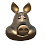### Copying vertices to Numpy arrays in Blender

If you want to do a lot of mathematical work on very large Blender meshes using plain Python might be too slow. Fortunately Blender comes bundled with Numpy which allows for fast calculations on vast arrays of numerical data.
To use Numpy you would need to copy for example vertex coordinates first before performing any calculations and then copy the results back again. This consumes extra memory and time. You wouldn't do this for a single operation like determining the average position of all vertices because Python can perform this so efficiently that it wouldn't compensate the extra setup time. However, when calculating many iterations of some erosion algorithm this might be well worth the cost.
In order to help in deciding whether this setup cost is worth the effort I decided to produce some benchmark values. My aim was to find the fastest method from several alternatives and to produce actual timings. Now your timings will be different of course but the trends will be comparable and I have provided the source code for my benchmark programs on GitHub.

## results, copying to a Numpy array

First we have a look at how much time it takes to copy the vertex coordinates from a mesh to a Numpy array. Given a the array of vertices `me.vertices` and a Numpy array `verts` we have several options:
```# blue line
verts = np.array([v.co for v in me.vertices])

# red line
verts = np.fromiter((x for v in me.vertices for x in v.co),
dtype=np.float64)
verts.shape = (len(me.vertices), 3)

# orange line
verts = np.fromiter((x for v in me.vertices for x in v.co),
dtype=np.float64,
count=len(me.vertices)*3)
verts.shape = (len(me.vertices), 3)

# green line
verts = np.empty(count*3, dtype=np.float64)
me.vertices.foreach_get('co', verts)
verts.shape = shape```
The blue line represents the creating of a Numpy array from a list. Even with list comprehension this has the disadvantage of creating a intermediate copy, which is also a Python list. Apparently this a costly operation as this naive method performes the worst of all.
The red line shows a huge increase in performance as we use a generator expression to access the coordinates. This will not create an intermediate list but the result is a one dimensional array. Changing the shape of an array in Numpy involves no copying so is very cheap.
We can still improve a little bit on our timing by preallocating the size for the Numpy array. The result is shown with the orange line.
The green line shows the fastest option. Blender provides a fast method to access attributes in a property collection and this method wins hands down. (thanks Linus Yng for pointing this out)

## results, copying from a Numpy array

Copying data back from a Numpy array can also be done in more than one way, the most interesting ones are shown below:
```# blue line
for i,v in enumerate(me.vertices):
v.co = verts[i]

# green line
for n,v in zip(verts, me.vertices):
v.co = n

# light blue line
def f2(n,v):
v.co = n

for v in itertools.starmap(f2, zip(verts, me.vertices)): pass

# orange line
verts.shape = count*3
me.vertices.foreach_set('co', verts)
verts.shape = shape
```
The conventional methods perform not significantly different, but again Blenders built-in `foreach_set` method outperforms all other other options by a mile, just like its `foreach_get` counterpart.

## Discussion

The actual timings on my machine (a intel core i7 running at 4GHz) indicate that we can copy about 1.87 Million vertex coordinates per second from a Blender mesh to a Numpy array and about 1.12 Million vertex coordinates per second from a Numpy array to a Blender mesh using 'conventional methods'. The 50% difference might be due to the fact that the coordinate data in a Blender mesh is scattered over the internal memory (as compared to Numpy's contiguous block of ram) and writing to scattered memory incurs a bigger hit form cache misses and the like than reading from it. Note that a vertex location consists of three 64 bit floating point numbers.
Using `foreach_get` and `foreach_set` this performance is greatly improved to 13.8 Million vertex coordinates per second and 10.8 Million vertex coordinates per second respectively (on my machine).
Overall, with this performance this means that on my machine even a simple scaling operation on a million vertices might already be faster with Numpy, like shown in the first example below, which on my machine is about 7x faster (not that scaling is such a perfect example, chances are Blender's scale operator is faster but it gives you an idea how much there is to gain if even for simple operations that are not natively available in Blender.
```    # numpy code with fast Blender foreach
fac = np.array([1.1, 1.1, 1.1])
count = len(me.vertices)
shape = (count, 3)

fac = np.array([1.1, 1.1, 1.1])

verts = np.empty(count*3, dtype=np.float64)
me.vertices.foreach_get('co', verts)
verts.shape = shape
np.multiply(verts,fac,out=verts)
verts.shape = count*3
me.vertices.foreach_set('co', verts)
verts.shape = shape

# 'classic code'
fac = Vector((1.1, 1.1, 1.1))

for v in me.vertices:
v.co *= fac
v.co *= fac
v.co *= fac```

## Benchmark availability

The .blend file with code and instructions can be downloaded from my GitHub repository.

1.Have you tried vertices.foreach_get?

>>> vertices = D.objects['Cube'].data.vertices
>>> verts=np.zeros(len(vertices)*3)
>>> vertices.foreach_get('co',vests)

Of course you have to reshape but still

2.again a good point, if the additional precision isn't needed then indeed it makes no sense using it.

3.Can we use the foreach_set and foreach_get in images? I mean reading and writing pixel info.

1.I don't think so: the image attribute is not a bpy_prop_collection so doesn't have a foreach_get

2.But you can assign an array of floats to the pixels attribute, see http://blender.stackexchange.com/questions/643/is-it-possible-to-create-image-data-and-save-to-a-file-from-a-script

You might try if this could be a numpy array as well

4.detail: you could use verts.ravel() (to flatten) in the last foreach and not do verts.shape before